# Paraboloid

﻿
Paraboloid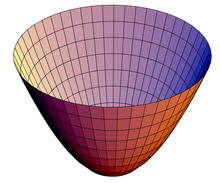Paraboloid of revolution

In mathematics, a paraboloid is a quadric surface of special kind. There are two kinds of paraboloids: elliptic and hyperbolic. The elliptic paraboloid is shaped like an oval cup and can have a maximum or minimum point. In a suitable coordinate system with three axes x, y, and z, it can be represented by the equation$\frac{z}{c} = \frac{x^2}{a^2} + \frac{y^2}{b^2}.$

where a and b are constants that dictate the level of curvature in the x-z and y-z planes respectively.

This is an elliptic paraboloid which opens upward.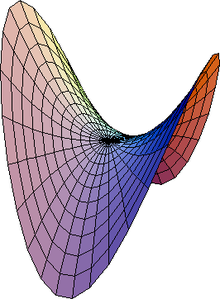Hyperbolic paraboloid

The hyperbolic paraboloid (not to be confused with a hyperboloid) is a doubly ruled surface shaped like a saddle. In a suitable coordinate system, a hyperbolic paraboloid can be represented by the equation$\frac{z}{c} = \frac{y^2}{b^2} - \frac{x^2}{a^2}.$

For c>0, this is a hyperbolic paraboloid that opens up along the x-axis and down along the y-axis (i.e., the parabola in the plane x=0 opens upward and the parabola in the plane y=0 opens downward).

## Properties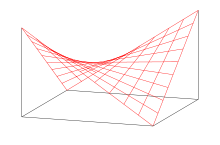The hyperbolic paraboloid is a doubly ruled surface, and thus can be used to construct a saddle roof from straight beams.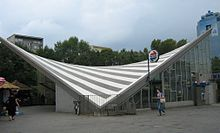Warszawa Ochota railway station , an example of a hyperbolic paraboloid structure.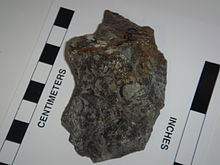The paraboloid shape of Archeocyathids creates conic sections on rock faces

With a = b an elliptic paraboloid is a paraboloid of revolution: a surface obtained by revolving a parabola around its axis. It is the shape of the parabolic reflectors used in mirrors, antenna dishes, and the like; and is also the shape of the surface of a rotating liquid, a principle used in liquid mirror telescopes. It is also called a circular paraboloid.

A point light source at the focal point produces a parallel light beam. This also works the other way around: a parallel beam of light incident on the paraboloid is concentrated at the focal point. This applies also for other waves, hence parabolic antennas.

The hyperbolic paraboloid is a doubly ruled surface: it contains two families of mutually skew lines. The lines in each family are parallel to a common plane, but not to each other.

## Curvature

The elliptic paraboloid, parametrized simply as$\vec \sigma(u,v) = \left(u, v, {u^2 \over a^2} + {v^2 \over b^2}\right)$$K(u,v) = {4 \over a^2 b^2 \left(1 + {4 u^2 \over a^4} + {4 v^2 \over b^4}\right)^2}$$H(u,v) = {a^2 + b^2 + {4 u^2 \over a^2} + {4 v^2 \over b^2} \over a^2 b^2 \left(1 + {4 u^2 \over a^4} + {4 v^2 \over b^4}\right)^{3/2}}$

which are both always positive, have their maximum at the origin, become smaller as a point on the surface moves further away from the origin, and tend asymptotically to zero as the said point moves infinitely away from the origin.

The hyperbolic paraboloid, when parametrized as$\vec \sigma (u,v) = \left(u, v, {u^2 \over a^2} - {v^2 \over b^2}\right)$

has Gaussian curvature$K(u,v) = {-4 \over a^2 b^2 \left(1 + {4 u^2 \over a^4} + {4 v^2 \over b^4}\right)^2}$

and mean curvature$H(u,v) = {-a^2 + b^2 - {4 u^2 \over a^2} + {4 v^2 \over b^2} \over a^2 b^2 \left(1 + {4 u^2 \over a^4} + {4 v^2 \over b^4}\right)^{3/2}}.$

## Multiplication table

If the hyperbolic paraboloid$z = {x^2 \over a^2} - {y^2 \over b^2}$

is rotated by an angle of π/4 in the +z direction (according to the right hand rule), the result is the surface$z = {1\over 2} (x^2 + y^2) \left({1\over a^2} - {1\over b^2}\right) + x y \left({1\over a^2}+{1\over b^2}\right)$

and if$\ a=b$ then this simplifies to$z = {2\over a^2} x y$.

Finally, letting$a=\sqrt{2}$, we see that the hyperbolic paraboloid$z = {x^2 - y^2 \over 2}.$

is congruent to the surface$\ z = x y$

which can be thought of as the geometric representation (a three-dimensional nomograph, as it were) of a multiplication table.

The two paraboloidal$\mathbb{R}^2 \rarr \mathbb{R}$ functions$z_1 (x,y) = {x^2 - y^2 \over 2}$

and$\ z_2 (x,y) = x y$

are harmonic conjugates, and together form the analytic function$f(z) = {1\over 2} z^2 = f(x + i y) = z_1 (x,y) + i z_2 (x,y)$

which is the analytic continuation of the$\mathbb{R}\rarr \mathbb{R}$ parabolic function$\ f(x) = {1\over 2} x^2.$

## Applications

The fluid in a spinning cylinder will form a paraboloid. This property can be used to make a liquid mirror telescope with a rotating pool of a reflective liquid, such as mercury, for the primary mirror.

The manufacturers of a widely-sold fried snack food, Pringles ersatz potato chips, utilize a hyperbolic paraboloid shape in order to produce a stackable snack.

Wikimedia Foundation. 2010.

### Look at other dictionaries:

• Paraboloïd — Paraboloïd, Fläche zweiter Ordnung, wie Ellipsoid und Hyperboloid. Man unterscheidet: 1) Das elliptische P. (Fig. 1), das keine Geraden enthält. Fig. 1. Elliptisches Paraboloid. Am einfachsten ist das Rotationsparaboloid, das durch Rotation einer …   Meyers Großes Konversations-Lexikon

• paraboloid — PARABOLOÍD, paraboloizi, s.m. (mat.) 1. Suprafaţă descrisă de o parabolă1 care se deplasează astfel încât planul ei rămâne mereu paralel cu el însuşi, iar vârful ei descrie o parabolă1 fixă. 2. (În sintagma) Paraboloid de rotaţie = suprafaţă… …   Dicționar Român

• paraboloid — [pə rab′əloid΄] n. a surface or solid formed so that sections parallel to the plane of symmetry are parabolas and sections perpendicular to it are ellipses (elliptic paraboloid), hyperbolas (hyperbolic paraboloid), or circles (paraboloid of… …   English World dictionary

• paraboloid — parabolòīd m <G paraboloída> DEFINICIJA mat. neograničena ploha bez centra simetrije, presjeci ravninama su parabole i druge konike [eliptički paraboloid; hiperbolički paraboloid] ETIMOLOGIJA grč. paraboloeidḗs: koji ukazuje na poređivanje …   Hrvatski jezični portal

• Paraboloid — Pa*rab o*loid ( loid), n. [Parabola + oid: cf. F. parabolo[ i]de.] (Geom.) The solid generated by the rotation of a parabola about its axis; any surface of the second order whose sections by planes parallel to a given line are parabolas. [1913… …   The Collaborative International Dictionary of English

• Parabolŏid — Parabolŏid, ist eine krumme nicht geschlossene Fläche, deren Gleichung zwischen rechtwinkligen Coordinaten ist az2 + by2 + cx2 + 2 d xy + 2 e xz + 2f yz + 2 gz + 2 hy + 2 ix + k = o unter der Bedingung abc – ad2 – be2 – cf2 + def = o. Man theilt… …   Pierer's Universal-Lexikon

• Paraboloid — Paraboloid, s. Flächen, Bd. 4, S. 60 …   Lexikon der gesamten Technik

• Paraboloid — Paraboloīd, eine krumme Fläche, deren Schnitt mit einer Ebene in gewissen Richtungen eine Parabel gibt. Durch Umdrehung einer Parabel um ihre Achse entsteht das Rotations P.; dasselbe gibt als Schnittkurven außer Parabeln noch Ellipsen und Kreise …   Kleines Konversations-Lexikon

• Paraboloid — Ein Paraboloid ist eine Fläche 2. Ordnung. Es entsteht durch das starre Entlangschieben einer Parabel entlang der anderen. Elliptisches Paraboloid …   Deutsch Wikipedia

• paraboloid — paraboloidal /peuh rab euh loyd l, par euh beuh /, adj. /peuh rab euh loyd /, n. Geom. a surface that can be put into a position such that its sections parallel to at least one coordinate plane are parabolas. Cf. elliptic paraboloid, hyperbolic… …   Universalium